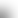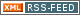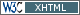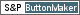www.LinuxHowtos.org howtos, tips&tricks and tutorials for linuxfrom small one page howto to huge articles all in one place

search text in:

Poll
Which linux distribution do you use?

poll results

Last additions:
using iotop to find disk usage hogs

using iotop to find disk usage hogs

words:

887

views:

149100

userrating:

average rating: 1.8 (88 votes) (1=very good 6=terrible)

May 25th. 2007:
Words

486

Views

245342

why adblockers are bad

Workaround and fixes for the current Core Dump Handling vulnerability affected kernels

Workaround and fixes for the current Core Dump Handling vulnerability affected kernels

words:

161

views:

128282

userrating:

average rating: 1.3 (28 votes) (1=very good 6=terrible)

April, 26th. 2006:

You are here: manpages

# MATH_ERROR

Section: Linux Programmer's Manual (7)
Updated: 2017-09-15
Index Return to Main Contents

## NAME

math_error - detecting errors from mathematical functions

## SYNOPSIS

```#include <math.h>
#include <errno.h>
#include <fenv.h>
```

## DESCRIPTION

When an error occurs, most library functions indicate this fact by returning a special value (e.g., -1 or NULL). Because they typically return a floating-point number, the mathematical functions declared in <math.h> indicate an error using other mechanisms. There are two error-reporting mechanisms: the older one sets errno; the newer one uses the floating-point exception mechanism (the use of feclearexcept(3) and fetestexcept(3), as outlined below) described in fenv(3).

A portable program that needs to check for an error from a mathematical function should set errno to zero, and make the following call

feclearexcept(FE_ALL_EXCEPT);

before calling a mathematical function.

Upon return from the mathematical function, if errno is nonzero, or the following call (see fenv(3)) returns nonzero

fetestexcept(FE_INVALID | FE_DIVBYZERO | FE_OVERFLOW |
FE_UNDERFLOW);

then an error occurred in the mathematical function.

The error conditions that can occur for mathematical functions are described below.

### Domain error

A domain error occurs when a mathematical function is supplied with an argument whose value falls outside the domain for which the function is defined (e.g., giving a negative argument to log(3)). When a domain error occurs, math functions commonly return a NaN (though some functions return a different value in this case); errno is set to EDOM, and an "invalid" (FE_INVALID) floating-point exception is raised.

### Pole error

A pole error occurs when the mathematical result of a function is an exact infinity (e.g., the logarithm of 0 is negative infinity). When a pole error occurs, the function returns the (signed) value HUGE_VAL, HUGE_VALF, or HUGE_VALL, depending on whether the function result type is double, float, or long double. The sign of the result is that which is mathematically correct for the function. errno is set to ERANGE, and a "divide-by-zero" (FE_DIVBYZERO) floating-point exception is raised.

### Range error

A range error occurs when the magnitude of the function result means that it cannot be represented in the result type of the function. The return value of the function depends on whether the range error was an overflow or an underflow.

A floating result overflows if the result is finite, but is too large to represented in the result type. When an overflow occurs, the function returns the value HUGE_VAL, HUGE_VALF, or HUGE_VALL, depending on whether the function result type is double, float, or long double. errno is set to ERANGE, and an "overflow" (FE_OVERFLOW) floating-point exception is raised.

A floating result underflows if the result is too small to be represented in the result type. If an underflow occurs, a mathematical function typically returns 0.0 (C99 says a function shall return "an implementation-defined value whose magnitude is no greater than the smallest normalized positive number in the specified type"). errno may be set to ERANGE, and an "overflow" (FE_UNDERFLOW) floating-point exception may be raised.

Some functions deliver a range error if the supplied argument value, or the correct function result, would be subnormal. A subnormal value is one that is nonzero, but with a magnitude that is so small that it can't be presented in normalized form (i.e., with a 1 in the most significant bit of the significand). The representation of a subnormal number will contain one or more leading zeros in the significand.

## NOTES

The math_errhandling identifier specified by C99 and POSIX.1 is not supported by glibc. This identifier is supposed to indicate which of the two error-notification mechanisms (errno, exceptions retrievable via fettestexcept(3)) is in use. The standards require that at least one be in use, but permit both to be available. The current (version 2.8) situation under glibc is messy. Most (but not all) functions raise exceptions on errors. Some also set errno. A few functions set errno, but don't raise an exception. A very few functions do neither. See the individual manual pages for details.

To avoid the complexities of using errno and fetestexcept(3) for error checking, it is often advised that one should instead check for bad argument values before each call. For example, the following code ensures that log(3)'s argument is not a NaN and is not zero (a pole error) or less than zero (a domain error):

double x, r;

if (isnan(x) || islessequal(x, 0)) {
/* Deal with NaN / pole error / domain error */ }

r = log(x);

The discussion on this page does not apply to the complex mathematical functions (i.e., those declared by <complex.h>), which in general are not required to return errors by C99 and POSIX.1.

The gcc(1) -fno-math-errno option causes the executable to employ implementations of some mathematical functions that are faster than the standard implementations, but do not set errno on error. (The gcc(1) -ffast-math option also enables -fno-math-errno.) An error can still be tested for using fetestexcept(3).

## SEE ALSO

gcc(1), errno(3), fenv(3), fpclassify(3), INFINITY(3), isgreater(3), matherr(3), nan(3)

info libc

## COLOPHON

This page is part of release 4.13 of the Linux man-pages project. A description of the project, information about reporting bugs, and the latest version of this page, can be found at https://www.kernel.org/doc/man-pages/.

## Index

NAME
SYNOPSIS
DESCRIPTION
Domain error
Pole error
Range error
NOTES
SEE ALSO
COLOPHON||- Powered by- Running on-
Copyright 2004-2020 Sascha Nitsch Unternehmensberatung GmbH::- Level Triple-A Conformance to Web Content Accessibility Guidelines 1.0 -
- Copyright and legal notices -
Time to create this page: 7.9 ms